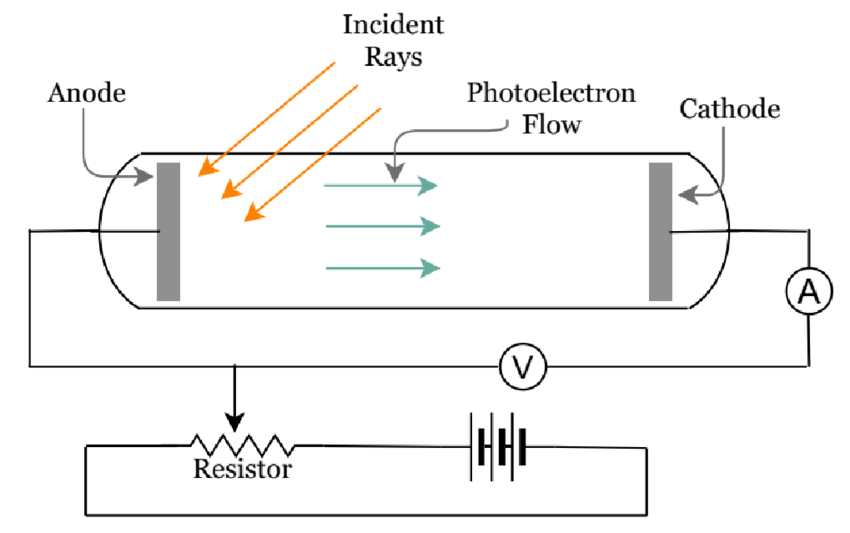# Photoelectric Effect Study Guide

INTRODUCTION

Stars are not spread about in space at random; instead, they form galaxies. Our Milky Way is the galaxy in which the Sun is located. However, how do you imagine astronomers studying these? The answer is the photoelectric effect. The faint light from the stars, which is too faint to be recognized and analyzed, is strong enough to fall on the photoelectric cell’s cathode and release electrons whose intensities and energy are understood as the intensities and temperatures of those stars.Source

## PHOTOELECTRIC EFFECT

• The definition of the photoelectric effect states that it is a phenomenon in which light causes electrons to be expelled from a metallic surface when exposed to the light of an appropriate frequency.
• The expelled electrons are called photoelectrons.
• The law of conservation of energy is the basic principle of the photoelectric effect.
• Albert Einstein was the first to successfully explain the laws of the photoelectric effect after years of research in this field.
• He came to the conclusion that this effect was caused by light energy being transported in discrete quantized packets called photons.
• A photoelectric effect diagram is given below.

## THE PHOTOELECTRIC EFFECT EXPERIMENT

• The photoelectric effect can be explained by the experiment wherein the electrons on the metal body absorb energy from the incident light and then use it to counteract the attractive forces that bind them to the metallic nuclei.
• The photons( the ‘particles’ of light that make up the visible spectrum) that strike the metal’s surface must have enough energy to overcome the attractive forces that bind the electrons to the nuclei in order for the photoelectric effect to occur.
• The threshold energy for the photoelectric effect is the least quantity of electricity required to remove an electron from a metal.Source

• The photoelectric effect experiment cannot be explained using the wave model of light.
• However, the particle nature of light, which can be viewed as a stream of electromagnetic energy particles, can explain this behavior.
• The photoelectric effect can be explained by Planck’s equation E = h𝜈 = hc/λ.

# SUMMARY

• The photoelectric effect is a phenomenon that causes electrons to be expelled from a metallic surface when exposed to the light of an appropriate frequency.
• The electrons at the surface of the metal absorb energy from the incident light and then use it to counteract the attractive forces that bind them to the metallic nuclei.
• The photoelectric effect can be explained by Planck’s equation:

E = h𝜈 = hc/λ.

## FAQs

Q. What is the photoelectric effect?

The photoelectric effect is a phenomenon that causes electrons to be expelled from a metallic surface when exposed to the light of an appropriate frequency.

Q. Give an example of the photoelectric effect?

The generation of electricity in solar cells is an example of the photoelectric effect.

Q. What is the reason for the photoelectric effect?

Conservation of mass is the main reason for the photoelectric effect.

Q. What is the charge of a photon?

A photon is a massless, stable particle with no electric charge.

We hope you enjoyed studying this lesson and learned something cool about the Photoelectric Effect! Join our Discord community to get any questions you may have answered and to engage with other students just like you! We promise, it makes studying much more fun!😎

## REFERENCE

1. Photoelectric Effect: https://flexbooks.ck12.org/cbook/ck-12-physics-flexbook-2.0/section/13.5/primary/lesson/photoelectric-effect-chem/ Accessed 14th April 2022.
2. Photoelectric Effect: https://www.britannica.com/science/photoelectric-effect Accessed 14th April 2022.
]]>